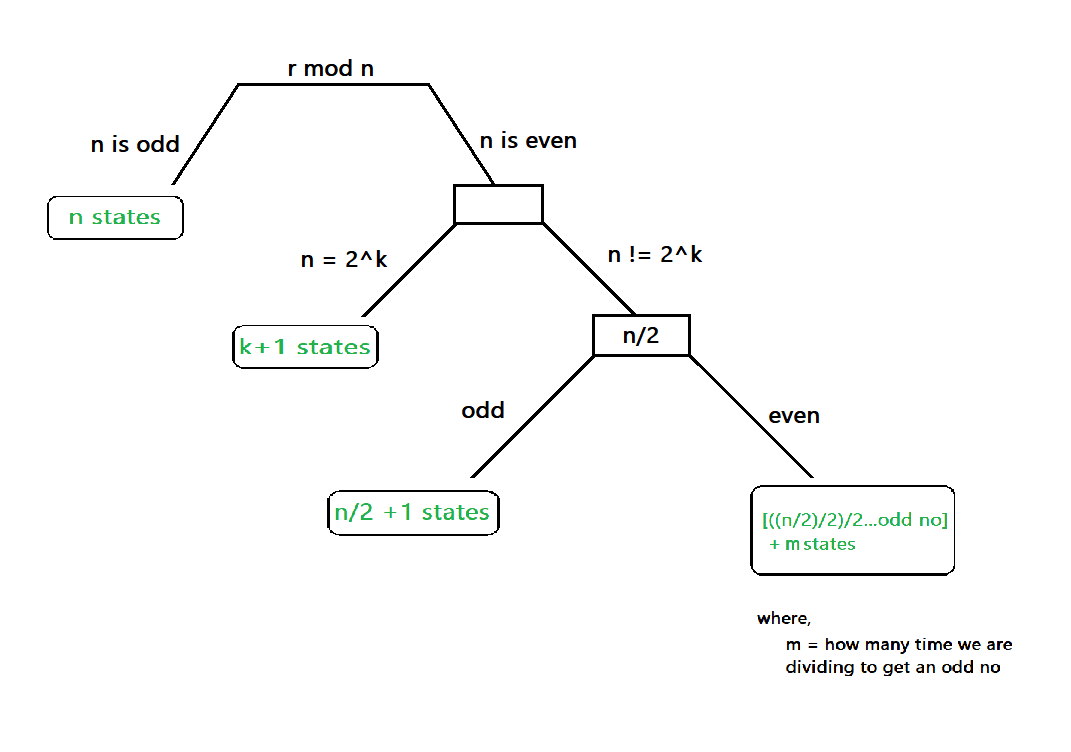Related Articles

# Short trick to find number of states in DFA that accepts set of all binary numbers which are mod by n

• Last Updated : 21 Sep, 2021

Suppose we have a question :

```Que: Construct minimal state DFA that accepts set of all binary no. which is 2 mod 5(say)
Ans: 5 states```

For solving these type of questions there is a traditional way of constructing the respective DFA for that problem. The problem in that traditional approach is that, it is time consuming and not all people can construct DFA perfectly in one go (which will lead to wrong answer).

So, here is a trick for solving these type of questions in just few seconds. Follow the steps shows in the diagram below  and after some practice it will be on your finger tips.STEPS:

1. If n is odd, then minimum of states will be n.
2. if n is not even:
• Check, if n is equal to the format 2^k (like 4 = 2^2, 8 = 2^3) , where k is any whole number.
• if n= 2^k. then minimum no of states will be k+1.
• But, if not n != 2^k
• Check if n/2 is odd, then minimum states will be n/2+1.
• If n/2 is even, then divide the no by 2 again and again till we get and odd digit , then add no of times the no was divided to get odd value [ ((n/2))/2….odd + m ]. The result of that sum will be the minimum no of states.
```EXAMPLE 1: 2 mod 5 where r=2,n=5
SOLUTION:
1. 5 is odd
Therefore, ans is 5 states (n states)```
```EXAMPLE 2: 4 mod 8 where r=4,n=8
SOLUTION:
1. 8 is even (so it can't be n states)
2. check n=2^k format
8=2^3
Therefore, ans is 4 states(k+1 states)```
```EXAMPLE 3: 10 mod 16 where r=10,n=16
SOLUTION:
1. 16 is even (so it can't be n states)
2. 16 != 2^k (so it can't be k+1 states)
3. n/2 is even (16/2=8, so it can't be n/2+1 states)
4. Divide n by 2 till we get odd no and keep a count on how many times we are dividing
16/2=8 , m=1
8/2=4 , m=2
4/2=2 , m=3
2/2=1 ,m=4   (odd found)
Therefore answer is 5 states (odd + m states)```

Attention reader! Don’t stop learning now.  Practice GATE exam well before the actual exam with the subject-wise and overall quizzes available in GATE Test Series Course.

Learn all GATE CS concepts with Free Live Classes on our youtube channel.

My Personal Notes arrow_drop_up